Technical Article

# Universal Logic Gates

November 06, 2015 by David Knight

## NAND and NOR gates are widely known to be universal logic gates, meaning that any other logic gate be made from NAND or NOR gates. This article discusses other universal logic gates.

### Introduction

A universal logic gate is a logic gate that can be used to construct all other logic gates.  There are many articles about how NAND and NOR are universal gates, but many of these articles omit other gates that are also universal gates. This article covers two input logic gates, demonstrates that the NAND gate is a universal gate, and demonstrates how other gates are universal gates that can be used to construct any logic gate.

### Two Input Logic Gates

For 2 logical inputs, there are 16 possible logic gates. Table 1 defines the 16 logic gates, shows logical symbol, behavior in symbolic logic, provides name, notes.

People are most familiar with the ones that are named: NAND, NOR, AND, OR, XOR. Table 1 includes these, as well as AND and OR gates with either input negated, logic 0 and logic 1(which aren't really logic gates), and some gates that aren't really binary.

 Output when AB is Gate # 00 01 10 11 Symbolic Logical Description Symbol Name/Description Note 0 0 0 0 0 0Always zero Not really a gate 1 0 0 0 1 A & BAND 2 0 0 1 0 A & ~BA and Not B Universal 3 0 0 1 1 AA Not BInary 4 0 1 0 0 ~A & BB and Not A Universal 5 0 1 0 1 BB Not Binary 6 0 1 1 0 (A & ~B) | (~A & B)XOR Also Not Equals Function 7 0 1 1 1 A | BOR 8 1 0 0 0 ~(A | B)NOR Universal 9 1 0 0 1 (A & B) | (~A & ~B)XNOR Also Equals Function 10 1 0 1 0 ~BNot B Not Binary 11 1 0 1 1 A | ~BA or Not B Universal 12 1 1 0 0 ~ANot A Not Binary 13 1 1 0 1 ~A | BB or Not A Unversal 14 1 1 1 0 ~(A & B)NAND Universal 15 1 1 1 1 1Always 1 Not really a gate

### NAND Gate is a Universal Gate

NAND gates can be connected to form any other logic gates. Figures 1,2,3 show how NAND gates can be connected to form INVERTER, AND, and OR gates. These gates can be combined to form the other logic gates according to the symbolic logic definitions in table 1.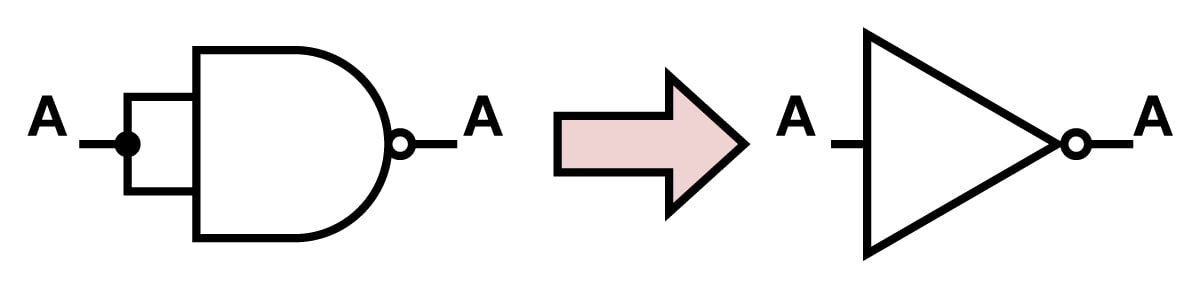Figure 1: Connecting a NAND gate to make an INVERTER gate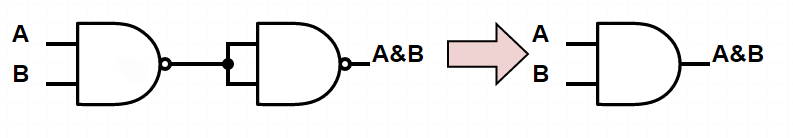Figure 2: Connecting NAND gates to make an AND gate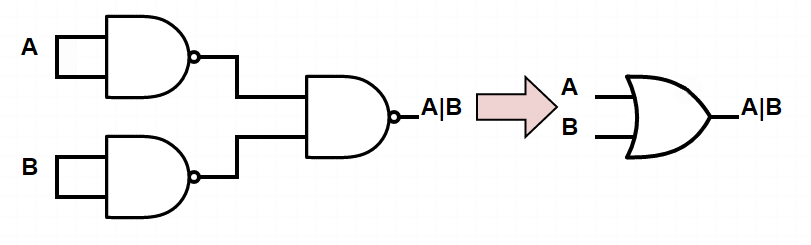Figure 3: Connecting NAND gates to make an OR gate

### Other Universal Gates

Gates 2,4,11, and 13 are universal gates. To prove this, we demonstrate building NAND gates with these gates. Gates 7 and 8 are the same gate but with different inputs inverted. Gates 9 and 10 are the same gate but with different inputs inverted. Proving that gates 2 and 11 are universal is enough to prove that gate 4 and 13 are universal as well.

Gate 11: A | ~B

Figures 4 and 5 show show gate 11 can be combined to create INVERTER and AND gates. INVERTER and AND gates can be connected in series to construct a NAND gate. Because gate 11 can be used to construct a NAND gate, and a NAND gate is a universal gate, gate 11 is a universal gate. Since gates 11 and 13 are mirrors of each other, gate 13 is also a universal gate.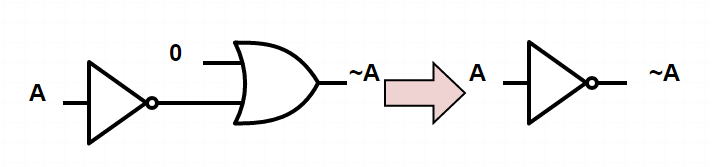Figure 4: Connecting gate 11(A |~B) to create an INVERTER gate.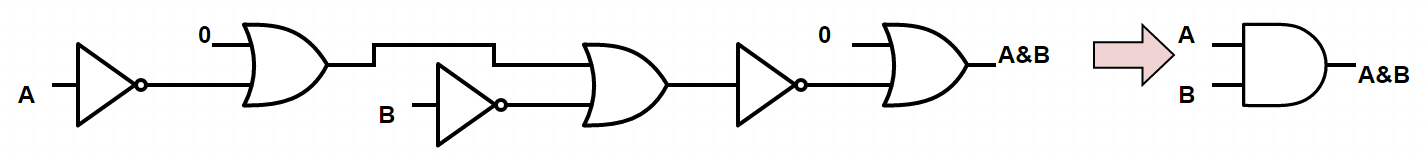Figure 5: Connecting gate 11(A |~B) to create an AND gate. There may be a way to do this with fewer gates. The figure here is the one the author could derive the quickest.

Gate 2: A & ~B

Figures 6 and 7 show show gate 2 can be combined to create INVERTER and AND gates. INVERTER and AND gates can be connected in series to construct a NAND gate. Because gate 2 can be used to construct a NAND gate, and a NAND gate is a universal gate, gate 2 is a universal gate. Since gates 2 and 4 are mirrors of each other, gate 4 is also a universal gate.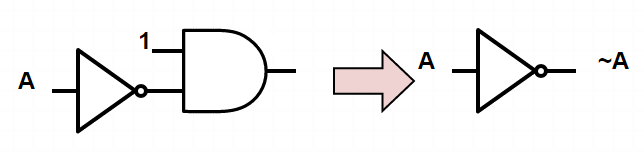Figure 6: Connecting gate 2(A &~B) to create an INVERTER gate.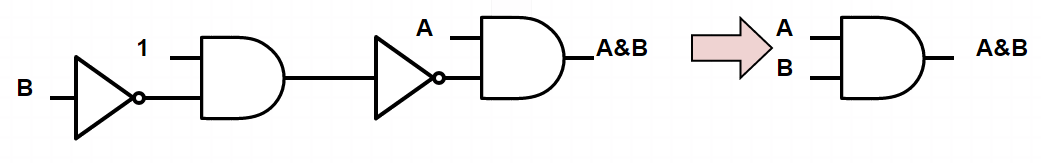Figure 7: Connecting gate 2(A &~B) to create an AND gate.

### Conclusion

This article has discussed logic gates, universal gates, demonstrated that the NAND gate is a universal gate, and proven that four additional universal logic gates exist. This is an interesting academic exercise. In practice, the author would recommend sticking to using NAND gates as universal gates because they are so cheap and small to construct, or if doing board level design using chips with the actual gates that are wanted and not using universal gates.• I
ilane November 13, 2015

loved the nixie tubes pic!

Like.
• C
Chandu csr July 21, 2017

It’s good if we remember the universal gates. But, if we don’t remember what they are exactly then it is not all going to be easy to realized .
Is there any trick that helps us to know whether the given gate is a universal gate?!!??

Like.
• M
MIS42N August 25, 2018
Two things to remember:  one connection (either input or output) must invert (turn a 0 into a 1, or a 1 into a 0).  the gate (ignoring the inversion) is either an OR or an AND gate.
Like.